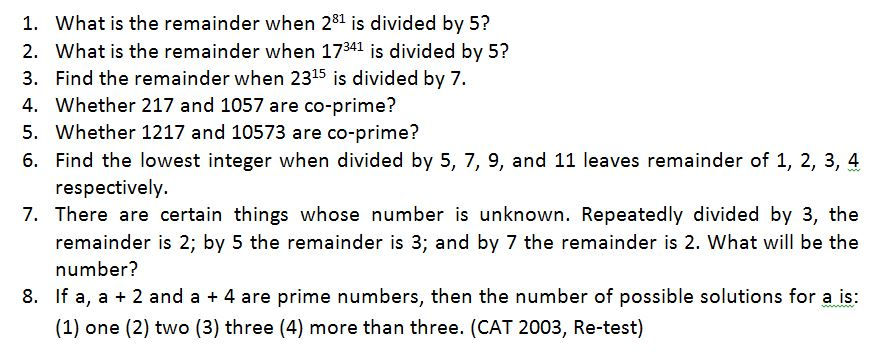top of page

# Modular Arithmetic (Congruent Modulo n)

Play with remainders

What a superb idea did Gauss have long time ago!!!! If you add two numbers, the remainders obtained after dividing the two numbers also get added up. For example:Similarly, if you subtract two numbers, the remainders obtained after dividing the two numbers also get subtracted. For example:Similarly, if you multiply two numbers, the remainders obtained after dividing the two numbers also get multiplied. For example:The same relation is not ALWAYS possible with division.

Modular Arithmetic and Congruence

We are already aware of four operations on integers. They are: addition, subtraction, multiplication and division. Congruent modulo n is a special kind of operation on integers which is in line with three operations stated above, i.e. addition, subtraction, and multiplication.

With this operation being at the central place, the Modular Arithmetic, invented by the famous Mathematician Carl Friedrich Gauss in 1801, is in fact a very elegant and useful tool in mathematics. This is a wonderful tool to solve problems like:

You are an aspirant of CAT/CLAT/CSAT (IAS). How would you solve these questions?To solve this type of questions within strict timelines, with precision and with accuracy you need a newer concept of arithmetic, better known as modular arithmetic.

Definition of Congruent Modulo n: For a positive integer n, two integers a and b are said to be congruent modulo n, if their difference (a – b) is divisible by a positive integer n, or in other words, is difference (a – b) is an integral multiple of n. We may say that there exists an integer k, such that a − b = kn.

This congruence relation is denoted by a ≡ b mod n. ……. (1)

(Note: For showing congruence relation, the signs == and ≡ have been used interchangeably.)

It was Gauss who came up with a clear-cut idea of congruence and notations to indicate the relationship among all integers that have a special property of leaving the same remainder when divided by a particular positive integer. This particular integer is called the modulus, and the arithmetic under the umbrella of this relationship is called the Modular Arithmetic.

The following example formalizes this concept.

For example, 33 == 7 mod 13 means that 33 and 7 are congruent modulo 13, because the difference between 33 and 7 is divisible by 13, and because both 33 and 7, when divided by 13, leave the same remainder, i.e. 7. Some other numbers with divisor being 7 are 2, 9, 16, .. We shall say these numbers are congruent to each other modulo 7, and write 16 == 9 == 2 (mod7).

###### Featured Posts
Check back soon
Once posts are published, you’ll see them here.